# 支持向量机学习之3-SVR（回归）ml 同时被 2 个专栏收录13 篇文章 1 订阅

Spark机器学习系列之13： 支持向量机SVM ：http://blog.csdn.net/qq_34531825/article/details/52881804

# ϵ−SVR $\epsilon-SVR$

SVR回归中，基本思路和SVM中是一样的，在 ϵSVR $\epsilon-SVR$[Vapnic,1995] 需要解决如下的优化问题。

min  12||w||2+Ci=1l(ξi+ξi)
s.t.  yi(wTxi+b)<ϵ+ξi(wTxi+b)yi<ϵ+ξiξi,ξi0

s.t.  yi(wTxi+b)<ϵ+ξi(wTxi+b)yi<ϵ+ξiξi,ξi0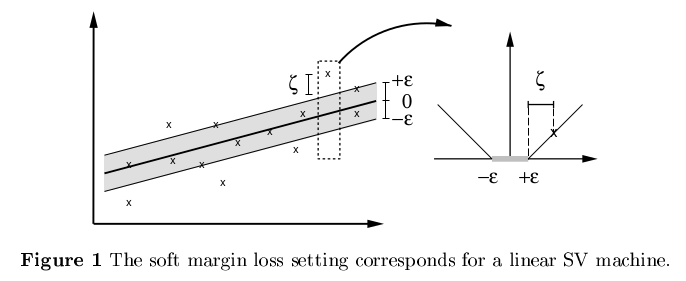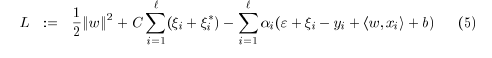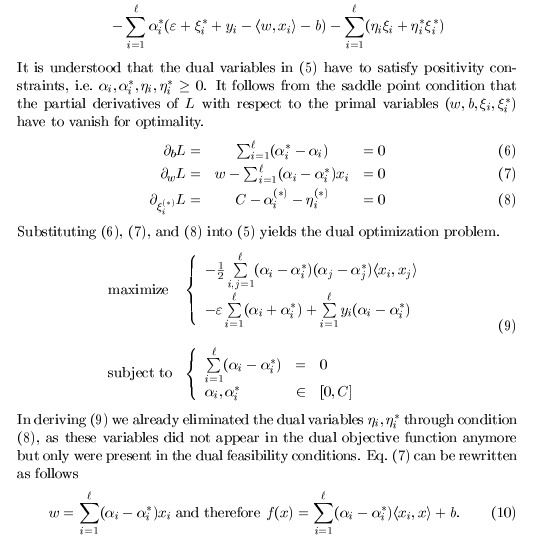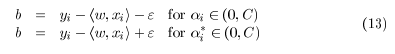# 使用核函数的 ϵ−SVR $\epsilon-SVR$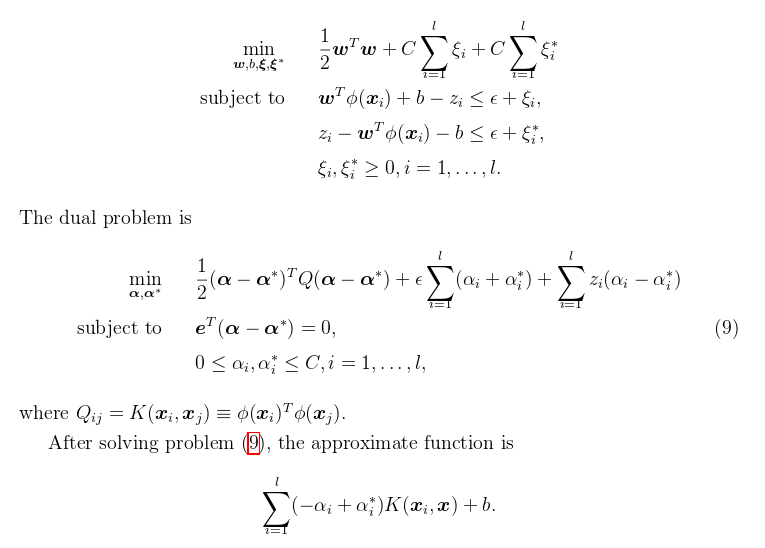# ν−SVR $\nu-SVR$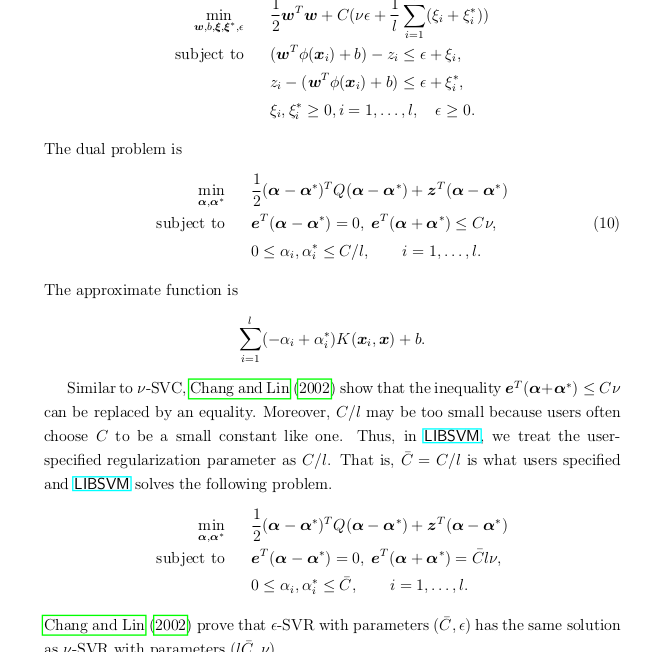Chang and Lin (2002) prove that ϵ $\epsilon$-SVR with parameters (C¯¯¯̄ ,ϵ) $( \overline C̄,\epsilon)$has the same solution as ν $\nu$-SVR with parameters (lC¯¯¯̄ ,ν) $(l\overline C̄,\nu)$.

# Scikit代码

#-*-coding:utf-8-*-
import numpy as np
from sklearn.svm import SVR
import matplotlib.pyplot as plt

###############################################################################
# Generate sample data
X = np.sort(5 * np.random.rand(40, 1), axis=0)  #产生40组数据，每组一个数据，axis=0决定按列排列，=1表示行排列
y = np.sin(X).ravel()   #np.sin()输出的是列，和X对应，ravel表示转换成行

###############################################################################
y[::5] += 3 * (0.5 - np.random.rand(8))

###############################################################################
# Fit regression model
svr_rbf10 = SVR(kernel='rbf',C=100, gamma=10.0)
svr_rbf1 = SVR(kernel='rbf', C=100, gamma=0.1)
svr_rbf1 = SVR(kernel='rbf', C=100, gamma=0.1)
#svr_lin = SVR(kernel='linear', C=1e3)
#svr_poly = SVR(kernel='poly', C=1e3, degree=3)
y_rbf10 = svr_rbf10.fit(X, y).predict(X)
y_rbf1 = svr_rbf1.fit(X, y).predict(X)
#y_lin = svr_lin.fit(X, y).predict(X)
#y_poly = svr_poly.fit(X, y).predict(X)

###############################################################################
# look at the results
lw = 2 #line width
plt.scatter(X, y, color='darkorange', label='data')
plt.hold('on')
plt.plot(X, y_rbf10, color='navy', lw=lw, label='RBF gamma=10.0')
plt.plot(X, y_rbf1, color='c', lw=lw, label='RBF gamma=1.0')
#plt.plot(X, y_lin, color='c', lw=lw, label='Linear model')
#plt.plot(X, y_poly, color='cornflowerblue', lw=lw, label='Polynomial model')
plt.xlabel('data')
plt.ylabel('target')
plt.title('Support Vector Regression')
plt.legend()
plt.show()

RBF不同参数：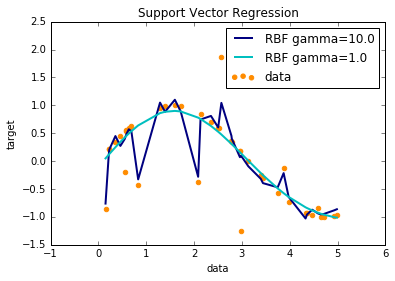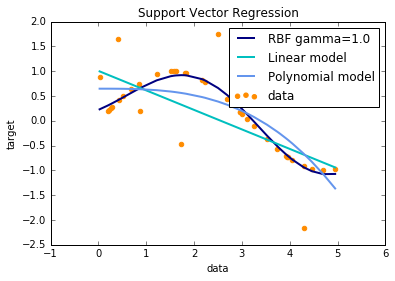（1）A Tutorial on Support Vector Regression ,Alex J
http://www.svms.org/regression/SmSc98.pdf
（2）LIBSVM:A library for Support Vector-Machines Chih-Chung Chang and Chih-Jen Lin
（3）支持向量机回归算法研究 硕士学位论文

03-3004-196万+
06-091万+
03-22238
03-132万+
08-08
04-176万+
12-211952
05-041万+
03-17629
03-141012
12-103828
06-142万+
09-024万+
04-23501
08-173万+
07-231156
12-29389
07-24点击重新获取扫码支付余额充值